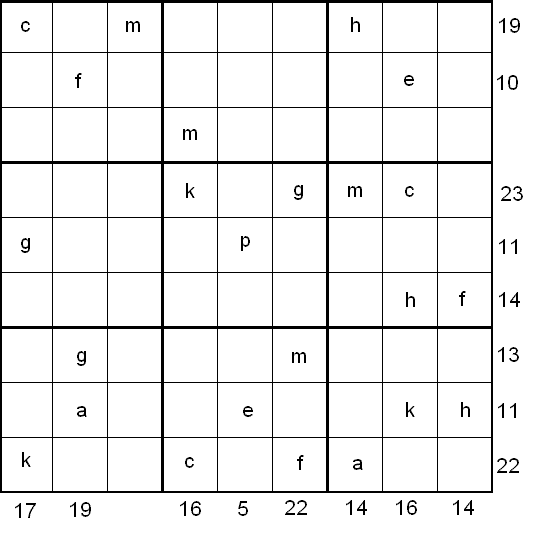You may also likeOverturning Fracsum

Solve the system of equations to find the values of x, y and z: xy/(x+y)=1/2, yz/(y+z)=1/3, zx/(z+x)=1/7Building Tetrahedra

Can you make a tetrahedron whose faces all have the same perimeter?Rudolff's Problem

A group of 20 people pay a total of £20 to see an exhibition. The admission price is £3 for men, £2 for women and 50p for children. How many men, women and children are there in the group?

Simultaneous Equations Sudoku

Age 11 to 16Challenge Level

by Henry KwokRules of Equation Sudoku

Like the standard sudoku, this sudoku variant has two basic rules:
1. Each column, each row and each box (3x3 subgrid) must have the numbers 1 through 9.
2. No column, row or box can have two squares with the same number.
3. The puzzle can be solved by finding the values of the 9 given variables in the squares of the 9x9 grid. At the bottom and right side of the 9x9 grid are numbers, each of which is the sum of a column or row of variables. Altogether a set of 16 equations can be formed from the columns and rows of variables and constants.
For example, in the first and second columns beginning from the left of the 9x9 grid, we can form the following equations:
c + g + k = 17
f + g + a = 19
In the fourth and fifth rows beginning from the top of the 9x9 grid, the following equations can be formed:
k + g + m + c = 23
g + p = 11

After solving all the equations, the puzzle is solved by the usual sudoku technique and strategy.